Order Form EM
Multiple patients, or group orders allowed.
Each patient must submit certification.
1 pound maximum per order.
•• Submit medical cannabis certification.
• - -
•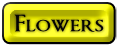•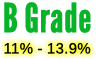•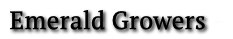\$2.11 to \$6.42 per gram
• Not for the seasoned user.
•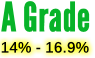•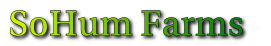• \$2.96 to \$6.57 per gram
•• \$2.58 to \$7.14 per gram
•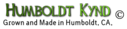• \$2.35 to \$6.57 per gram
•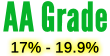•\$4.10 to \$10.00 per gram
•\$4.82 to \$9.28 per gram
•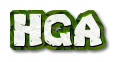• \$2.38 to \$7.14 per gram
•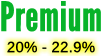•\$4.57 to \$10.71 per gram
• \$3.17 to \$10.00 per gram
• \$3.29 to \$10.00 per gram
•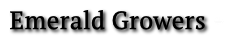\$4.57 to \$10.71 per gram
•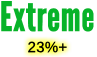\$5.04 to \$12.14 per gram
•• \$6.51 to \$11.42 per gram
••\$5.04 to \$12.14 per gram
•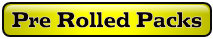• \$5.95 to \$8.21 each
• \$4.35 to \$6.78 each
• \$7.16 to \$9.28 each
• \$5.95 to \$8.21 each
• \$8.82 to \$14.50 per gram
• \$8.82 to \$14.50 per gram
•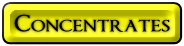•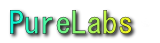• \$3.98 to \$10.71 gram
• \$7.15 to \$15.00 gram
• \$8.25 to \$15.71 gram
• \$5.72 to \$15.00 gram
•• \$5.72 to \$15.00 gram
• \$5.73 to \$15.71 gram
• \$6.86 to \$16.42 gram
• \$5.72 to \$15.00 gram
•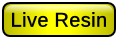One Ounce Jars
•\$17.35 to \$35.00 per gram
• \$42.50 to \$53.33 per gram
• \$42.50 to \$53.33 per gram
• \$42.50 to \$53.33 per gram
• \$42.50 to \$53.33 per gram
•• \$10.89 to \$16.42 per gram
•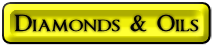•• \$40.00 to \$55.00 per gram
•• \$44.33 to \$75.00 per gram
•• \$7.15 to \$15.00 gram
• \$23.12 to \$40.00 gram
•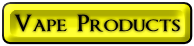•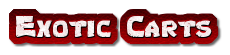\$12.95 to \$23.33 per gram
• At least 2 of each flavor.
• \$6.25 to \$12.00 per gram
•\$22.85 to \$41.66 gram
•• One gram oil each
• One gram oil each
• One gram oil each
• One gram oil each
• One gram oil each
• One gram oil each
• Vape Pen with charger
••\$19.70 To \$33.33 per gram
•\$22.85 to \$41.66 gram
•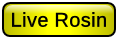\$22.85 to \$41.66
•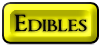•• \$3.65 to \$5.37 each
• \$4.48 to \$6.12 each
• \$22.59 to \$30.50 each
• \$0.88 cents to \$1.42 gram
• \$1.28 to \$1.82 gram
• \$3.00 to \$4.60 each
•• \$12.25 to \$17.00 each
• \$9.65 to \$22.50 each
• \$9.65 to \$22.50 each
• \$9.65 to \$32.50 each
• \$14.05 to \$37.50 each
• \$14.05 to \$37.50 each
• \$14.10 to \$38.00 each
• \$17.35 to \$39.00 each
• \$17.35 to \$39.00 each
• \$17.35 to \$39.00 each
• \$17.35 to \$39.00 each
• \$17.35 to \$39.00 each
• \$17.35 to \$39.00 each
• \$17.35 to \$39.00 each
• \$19.30 to \$39.50 each
• \$19.30 to \$39.50 each
• \$4.93 to \$7.00 per gram
• \$4.93 to \$7.00 per gram
•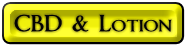•• \$2.53 to \$4.10 per gram
• \$2.53 to \$4.10 per gram
• \$2.53 to \$4.10 per gram
•• \$0.68 cents to \$1.16 cents per gram

•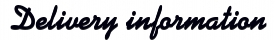• ### All orders must be paid in full in advance.

Depending upon your location, delivery time varies from 2, up to 5 days, in extreme cases, or delays from extreme weather conditions.
• *USPS money order may delay delivery up to 2 days.
They can be difficult to cash.
• Total weight